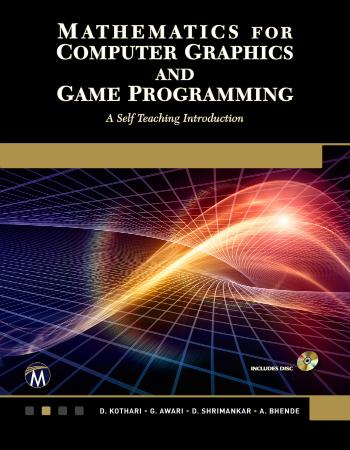## Mathematics For Computer Graphics And Game Programming - A Self-Teaching IntroductionMathematics For Computer Graphics And Game Programming - A Self-Teaching Introduction
pdf | 15.28 MB | Author :Kothari, D. P.;Awari, G.;Shrimankar, D.;Bhende, A.;

Book Description :

Designed to explain the mathematical concepts involved in computer graphics and its entities, this book is ideal for courses in computer graphics, engineering, game development, as well as for professionals in industry. It begins with simple concepts such as how an image is generated on the screen and then moves to cover the different algorithms for the generation of simple geometry on the screen. The subsequent chapters include two-dimensional and three-dimensional transformations, parametric representation of planar curves and parametric representation of space curves such as cubic splines, Bezier curves, etc. In addition to programming in C, OpenGL, and several other topics, it includes a final chapter on the methods of generating 3D models.

Selected Topics
Introduction to Computer Graphics • Vector Representation of Geometric Entities • Two-dimensional Transformation • Three-dimensional Transformation • Parametric Representation of Planar Curves • Parametric Representation of Space Curves • Parametric Representation of Surfaces • Windowing and Clipping • Generation of 3D Models • Projections • Graphics Programs in the C Language • OpenGL with Computer Graphics • Programming Graphics Using OpenGL. Appendices. Index.

Features
+Covers generation of 3D models and parametric representation of space curves
+Includes chapters on programming graphics in C and OpenGL

Category :